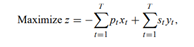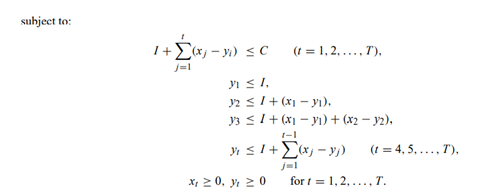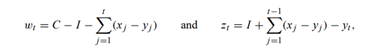# For the minimum-cost flow model given in Exercise 22, with the optimal solution determined in Exercise 23, answer

For the minimum-cost flow model given in Exercise 22, with the optimal solution determined in Exercise 23, answer the following questions.

a) For each no basic variable, determine the range on its objective-function coefficient so that the current basis remains optimal.

b) For each basic variable, determine the range on its objective-function coefficient so that the current basis remains optimal.

c) Determine the range on each righthand-side value so that the basis remains unchanged.

d) In question (c), the righthand-side ranges are all tight, in the sense that any change in one righthand-side value by itself will apparently change the basis. What is happening?

Q148. The following model represents a simple situation of buying and selling of a seasonal product for profit:In this formulation, the decision variables xt and yt denote, respectively, the amount of the product purchased and sold, in time period t. The given data is:

pt = per unit purchase price in period t,

s= per unit selling price in period t,

I = amount of the product on hand initially,

C = capacity for storing the product in any period.

The constraints state (i) that the amount of the product on hand at the end of any period cannot exceed the storage capacity C, and (ii) that the amount of the product sold at the beginning of period t cannot exceed its availability from previous periods.

a) Letdenote slack variables for the constraints (with z1 = I − y1). Show that the given model is equivalent to the formulation on page 363.

b) State the dual to the problem formulation in part (a), letting ui denote the dual variable for the constraint +xi , and vj denote the dual variable for the constraint containing +y.

c) Into what class of problems does this model fall? Determine the nature of the solution for T = 3.